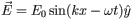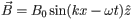# Problem: ,.This wave is linearly polarized in the y-direction. a. What is the wavelength λ of the wave described in the problem introduction?b. What is the period T of the wave described in the problem introduction?c. What is the velocity v of the wave described in the problem introduction?

###### FREE Expert Solution

Propagation constant:

$\overline{){\mathbf{k}}{\mathbf{=}}\frac{\mathbf{2}\mathbf{\pi }}{\mathbf{\lambda }}}$

Period:

$\overline{){\mathbf{\omega }}{\mathbf{=}}\frac{\mathbf{2}\mathbf{\pi }}{\mathbf{T}}}$

(a)

λ = 2π/k

80% (91 ratings)###### Problem Details,.

This wave is linearly polarized in the y-direction

a. What is the wavelength λ of the wave described in the problem introduction?

b. What is the period T of the wave described in the problem introduction?

c. What is the velocity v of the wave described in the problem introduction?